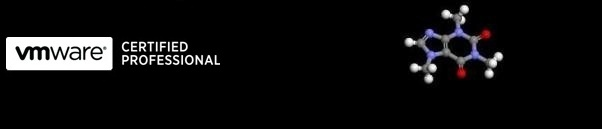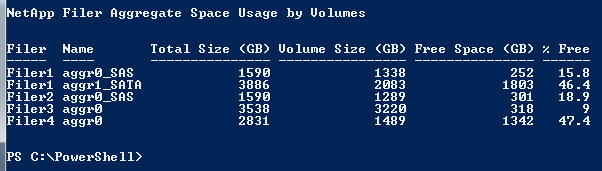...hopefully some useful VMware related stuff

# NetApp filer Aggregate space used by Volumes report

19/06/2010 12:00

To avoid over-committing storage it is essential to know how much space you have assigned to volumes on your aggregates. If you just run an aggregate list, the amount of space shown as used and free doesn't equal the actual amount used.

The script below creates a table showing Filer, the aggregate name, the total aggregate size and then the total of all the volumes on the aggregate, then a calculation of free space and percent free. An example is shown below:# NetApp Aggregate Storage Space Usage
# https://www.a2-alpha.co.uk
# 20100619
# 1.0

\$filers = @()
\$filers += "Filer1"
\$filers += "Filer2"
\$filers += "Filer3"
\$filers += "Filer4"
\$us = "root"
Clear
""
"Collecting information..."
""
\$AggrReport = @()
ForEach(\$filer in \$filers)
{
\$AggrTable = @()
ForEach(\$Aggregate in (Get-NaAggr))
{
\$AggrVolSizeAgTotal = 0
ForEach(\$AggrVol in Get-NaVol | where {\$_.ContainingAggregate -eq \$Aggregate.Name})
{
\$AggrVolSizeAgTotal += \$AggrVol.SizeTotal
}
\$AggrVolSizeAgTotal = [Math]::Round((\$AggrVolSizeAgTotal/1024/1024/1024),0)
\$AggrSizeGB = [Math]::Round(((\$Aggregate.SizeTotal)/1024/1024/1024),0)
\$AggrName = \$Aggregate.Name
\$AggrObj = "" | Select Filer, Name, "Total Size (GB)", "Volume Size (GB)", "Free Space (GB)", "% Free"
\$AggrObj.Filer = \$filer
\$AggrObj.Name = \$AggrName
\$AggrObj."Total Size (GB)" = \$AggrSizeGB
\$AggrObj."Volume Size (GB)" = \$AggrVolSizeAgTotal
\$AggrObj."Free Space (GB)" = \$AggrSizeGB - \$AggrVolSizeAgTotal
\$AggrObj."% Free" = [Math]::Round((((\$AggrObj."Free Space (GB)")/(\$AggrSizeGB))*100),1)
\$AggrTable += \$AggrObj
}
Disconnect-NaServer
\$AggrReport += \$AggrTable
}
clear
""
"NetApp Filer Aggregate Space Usage by Volumes"
""
\$AggrReport | ft -autosize